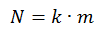# Testing if Number is Prime

by Zoran Horvat
Jan 06, 2014

## Problem Statement

Given an integer number N, N > 1, write a function which returns true if that number is prime and false otherwise. Number is prime if it has no other divisors than 1 and itself.

Example: For numbers 2, 5, 17 function should return true. For number 143 function should return false because that number is divisible by 11 and 13.

## Problem Analysis

We can discover that a given number is prime by failing to prove the opposite, i.e. by verifying that there is no eligible value which divides it without remainder. The trick in this task is to properly identify eligible divisors. These should be numbers greater than one which are not equal to the number tested. Further on, there is no point in taking into account values greater than N because none of them could possibly divide N. So, by now we have constrained candidates to set {2, 3, ..., N-1}.

Now suppose that there is such a value k which is the smallest number which divides N:This implies that m is also a divisor of N. But the fact that k is the smallest divisor implies that m cannot be less than k. From these facts we can derive one important conclusion:This has significantly reduced the problem. But we can make a step further. There is no point in trying to divide N with even values of k, except the trivial candidate 2. This because if N is not divisible by 2, then there is no chance that any other even number could divide it. The same logic goes with number 3. If 3 doesn't divide N, then none other multiple of 3 can divide N either. This leads to an interesting conclusion about possible divisors of N: the only viable candidates that could divide N are values 2, 3 and odd numbers around multiples of 6:This opts out roughly two out of three candidates not exceeding square root of N. And here is the pseudocode which solves the problem:

``````function IsPrime(n)
begin
result = false
if n <= 3 then
result = true
else if n mod 2 <> 0 AND n mod 3 <> 0 then
begin
k = 5
step = 2
while k * k <= n AND n mod k <> 0
begin
k = k + step
step = 6 - step
end

if n = k OR n mod k <> 0 then
result = true
end
return result
end
``````

## Implementation

The following C# code is a console application which implements the IsPrime function to test whether its argument is prime or not:

``````using System;

{

public class Program
{

static bool IsPrime(int n)
{

bool result = false;

if (n <= 3)
{
result = true;
}
else if (n % 2 != 0 && n % 3 != 0)
{

int k = 5;
int step = 2;

while (k * k <= n && n % k != 0)
{
k = k + step;
step = 6 - step;
}

if (n == k || n % k != 0)
result = true;

}

return result;

}

static void Main(string[] args)
{

while (true)
{

Console.Write("Enter number (zero to exit): ");

if (n <= 0)
break;

if (IsPrime(n))
Console.WriteLine("Number {0} is prime.", n);
else
Console.WriteLine("Number {0} is not prime.", n);

}

}

}
}
``````

## Demonstration

When application above is run, it produces the following output:

``````Enter number (zero to exit): 2
Number 2 is prime.
Enter number (zero to exit): 3
Number 3 is prime.
Enter number (zero to exit): 17
Number 17 is prime.
Enter number (zero to exit): 18
Number 18 is not prime.
Enter number (zero to exit): 143
Number 143 is not prime.
Enter number (zero to exit): 64657551
Number 64657551 is not prime.
Enter number (zero to exit): 64657553
Number 64657553 is prime.
Enter number (zero to exit): 0
``````